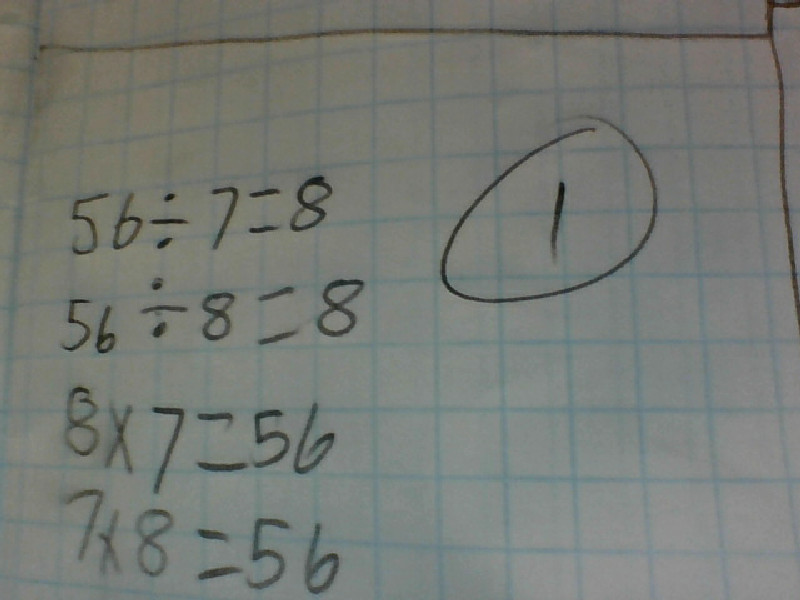## Wednesday, 27 May 2015

### Term 2 week 6 Exponents follow up 2015

Walt: Use more than one strategy to solve multiplication number problemsThis is how I got my by using division and multiplication. 56 divide by 7=8
56 divide by 8=7 and then 8x7=56 7x8=56. That's how I got my answer.

#### 1 comment:

1.